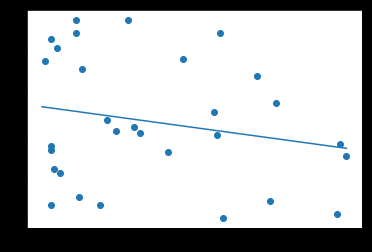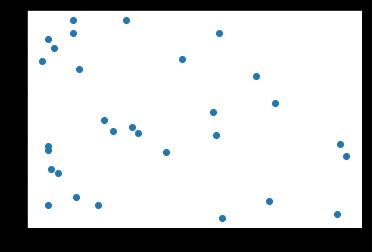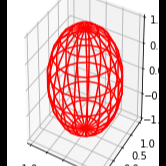How to make a linear regression FROM SCRATCH(not scratch scratch) 😎💻(machine learning)(python)

# why I'm writing this tutorial

😎💻 Last to last year I first started with machine learning. I made a neural net in java script to take in random inputs and give out random outputs. In the course of a month I learnt how to make neural nets that actually work with real data. I didnt understand a thing about how they worked. All I knew was what worked and what didnt. I ran everything on intuition. But thats not how anyone should start. We should start by understanding the basics. For me, the first time I ever made a machine learning algorithm from scratch was when I made a linear regression

# what is machine learning and linear regression?

What I said above might not make any sense if you dont read this. If you already know what machine learning is, skip this.

Now machine learning is when a program can do something without having to be told to do it explicitly using `if``else` statements.

Suppose you have a database with,lets say - one million records. This database gives you the details of one million people. Details about their entire life (tests, colleges they have gone to, jobs, crime they have comitted in the past) and whether they have gotten a job in a company. You are an employer and you have been asked to make sense of all this and make a program to take these details in and say whether they should join the company or not.If you use if else statements for all these parameters its going to take a lot of time since you also have to go through the data and you will probably end up with RSI and depression due to unemployment. machine learning solves this. It analyses the data and then predicts on other data (people applying for the job now).

The above example will need very sophisticated methods of analysis and we arent going to learn that in this tutorial. What we are going to learn is a much simpler and easier to understand at first concept - linear regression.

Remember grade 7 (or was it only taught in grade 7 for us? ) when the teacher gave you these points on a graph and told you to draw the "line of best fit" ? That's what linear regression does. A human eye can make a line of best fit quite easily by just looking at the graph, but that's often not very accurate. We are going to draw that same line of best fit with python and its going to be wayyyy more accurate.tl;dr we are going to draw a line of best fit on random data with python because why not

# The code and explanation (to the extent I can explain it)

If you haven't learnt python yet, dont worry, if you know a programming language its probable that you will understand python without having to learn it. After you're done if you implement this other languages then comment down the code and if you want I can include them in this tutorial itself :)

## what are we going to import?

In most tutorials on machine learning they import a lot of packages, enough to discourage people from learning about it. I don't want this to be like that so we are going to import as few packages as possible at the expense of writing more code for the tasks they could have accomplished faster. This will also give you a sense of what happens really in the code, not just "oh, it works!".

so after all that explanation here are the imports:

``````from random import randint
import matplotlib.pyplot as plt
import math``````
• I have imported the `random` library for the `randint` function (we will be generating random data)
• The `matplotlib` library is there to visualize the random data we create.Graphing libraries are quite helpful in machine learning.
• I have also imported the `math` library for the square root function.

## any kind of variables we need to be declared ✔🤢🥽🔏

I like declaring all the variables at the beginning when I can because umm... DONT QUESTION ME ITS WHAT I LIKE OK? I FEEL SAD NOW THAT U JUDGE ME!

so here are the variables:

``````mx=0
my=0
sy=0
sx=0
r=0
m=0
c=0
y=[]
x=[]
xdev=[]
ydev=[]
xydev=[]
xdevsquare=[]
ydevsquare=[]``````

And this is what each of them does :

• `mx` - mean of x ( integer/float value ,scalar)
• `my` - mean of y (integer/float value,scalar)
• `sx` - standard deviation of x ( integer/float value,scalar)
• `sy` - standard deviation of y ( integer/float value,scalar)
• `r` - Pearson's correlation ( integer/float value,scalar)
• `m` - slope (A.K.A "w1"/"weight1") of linear regression line ( integer/float value,scalar)
• `c` - y intercept (A.K.A "model bias") of line ( integer/float value,scalar)
• `x` - x values in the data (integer/float values,array)
• `y` - y values in the data (integer/float values,array)
• `xdev` - x deviation values (integer/float values,array)
• `ydev` - y deviation values (integer/float values,array)
• `xydev` - x deviation values * y deviation values (integer/float values,array)
• `xdevsquare` - x deviation values squared (integer/float values,array)
• `ydevsquare` - y deviation values squared (integer/float values,array)

## data generation 😎

Now the fun begins!

In this we are appending some random values to x and y using the `randint(start,stop)` function.Our values are ranging from 0 (start) to 100 (stop). You can change these values if you like, and see what happens. If you want, you can also make your own x and y values ( provided they have the same length... )

``````start=0
stop=100
for i in range(30):
x.append(randint(start,stop))
y.append(randint(start,stop))``````

Ok you got me! I dont declare ALL variables at the start. Hey I tried OK?

### Now to visualize the data we just created:

to visualize the data we are going to make the scatter graph we write the following code:

``plt.scatter(x,y)``

To show it we write:

``plt.show()``

here is the graph I got:## The calculations!!😎💻🖊🧮

• This is the tricky bit! Buckle up! get ready for a rocky ride!

### mean of X

First we find `mx`, the mean of x. We do this by adding all the x values to `mx` then dividing it by the length of `x`

``````for i in x:
mx+=i
mx/=len(x)
mx``````
• for non python users, /= means mx = mx/len(x)

### mean of y

Here we find the mean of `y` -> `my`

``````for i in y:
my+=i
my/=len(y)
my``````

### deviation of x

deviation is the value of x deviating or not being the same as the mean of x

Now is the time to find the deviations of x from the mean. We will store all of these in the variable `xdev`. We find each deviation by subtracting `mx` from `x` values. The syntax at the end, `xdev[:4]` simply gives the output of only four items from its array, instead of giving too many.

``````for i in x:
xdev.append(i-mx)
xdev[:4]``````

### deviations of y

• we do the very same thing with the y values
``````for i in y:
ydev.append(i-my)
ydev[:4]``````

### multiply the deviations of x and y

• We now multiply the `xdev` and `ydev` values together . `xdev``ydev`
``````for i in range(len(xdev)-1):
xydev.append(xdev[i]*ydev[i])
xydev[:4]``````

### square of x's deviation

Now we find the square of `xdev`

``````for i in xdev:
xdevsquare.append(i**2)
xdevsquare[:4]``````

### square of y's deviation

Same thing with y

``````for i in ydev:
ydevsquare.append(i**2)
ydevsquare[:4]``````

### standard deviation of x

we now find the standard deviation of x using the standard deviation formula.The formula says that we have to subtract the mean of x from x and square that.Then square root the value that comes and divide it by the length of x.If you dont understand this explanation then search it up on the web!

``````for i in x:
sx+=(math.sqrt((i-mx)**2))
sx/=len(x)
sx``````

### standard deviation of y

``````for i in y:
sy+=(math.sqrt((i-my)**2))
sy/=len(y)
sy``````

### correlation of the data

Data can be positvely correlated meaning the trend in the data is positive. I hope that explains it. If not, I'm not the best teacher for it, you can search up data correlation and see some videos maybe on it to get a better grasp of it
Now we find the correlation of the data.We do this using the formula for pearsons correlation. In it you have to divide the sum of all the `xydev` values by the square root of the multiplication of the sum of `xdevsquare` and `ydevsquare`. This is the formula:``````xy_sum=0
xsquare_sum=0
ysquare_sum=0
for i in range(len(x)-1):
xy_sum+=xydev[i]
xsquare_sum+=xdevsquare[i]
ysquare_sum+=ydevsquare[i]
r=xy_sum/(math.sqrt(xsquare_sum*ysquare_sum))``````

### the gradient of the line

If I havent said this earlier, I might as well say it now, the line of best fit is a line. That means we can describe it using a formula - `y = mx + c`. `m` is for gradient and `c` is for the y intercept.

The way to find `m` is by multiplying `r`,the correlation by the division of `sy` and `sx`

``m = r*sy/sx``

### find c

now we find the y intercept, c

to find c, you have to subtract `m` multiplied by `mx` from `my`

``c = my - (m*mx)``

## visualizing what you

THE BORING STUFF IS OVER NOW (unless ur a big nerd and found all that fun)

THIS IS THE BEST PART. IF UR FELING LIKE GOING TO THE LOO OR FEELING THIRSTY OR HUNGRY GO DO WHAT U GOT TO DO. YOU DON'T WANT TO MESS THE BEAUTY OF WHAT IS NOW GOING TO BE SHOWN TO YOU. YOU DON'T WANT TO DO THAT TRUST ME. IF YOU ARE FEELING NAUSEATED BY MY TUTORIAL THEN GO AND VOMIT.NOTHING CAN STAND BETWEEN YOU AND THIS LOVELY BIT

Y is predicted y and X is x\

``````Y=[]
X=[]
for i in range(stop):
Y.append((m*i)+c)
X.append(i)
plt.scatter(x,y)
plt.plot(X,Y)
plt.show()``````

Now here is the result I got (everyone's results will be different because of that random data), and you cant easily see the correlation either(again, random data) I could have done a better job on the data production with with the numpy library but never mind that# prerequisites

this is at the end because why not?

• you should have a good understanding of programming
• you should have a good understanding of basic linear algebra
• Its nice if you know a bit of statistics
if you have any doubts, any, no matter how dumb they might seem to you, they arent to me, so please ask whatever you want(on this topic) in the comments section :)

# the end:

I hope my markdown isnt too bad, I worked for long on this tutorial. Here is a colab link, its nice for all this kind of programming or you can also do it on replit which is better since I can help out. Here is the link to google colab anyways tho - https://colab.research.google.com

tl;dr we made an amazing linear regression line and since (if) you didnt read the entire thing, you aint getting none of that code!